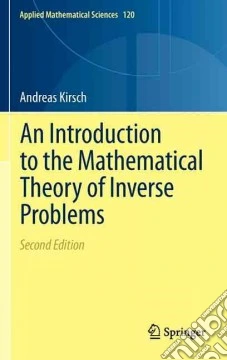ricerca
avanzata

# An Introduction to the Mathematical Theory of Inverse Problems

## Un libro in lingua di Andreas Kirsch edito da Springer Verlag, 2011

• € 77,10
• Il prezzo è variabile in funzione del cambio della valuta d’origineThis book introduces the reader to the area of inverse problems. The study of inverse problems is of vital interest to many areas of science and technology such as geophysical exploration, system identification, nondestructive testing and ultrasonic tomography. The aim of this book is twofold: in the first part, the reader is exposed to the basic notions and difficulties encountered with ill-posed problems. Basic properties of regularization methods for linear ill-posed problems are studied by means of several simple analytical and numerical examples.The second part of the book presents two special nonlinear inverse problems in detail - the inverse spectral problem and the inverse scattering problem. The corresponding direct problems are studied with respect to existence, uniqueness and continuous dependence on parameters. Then some theoretical results as well as numerical procedures for the inverse problems are discussed. The choice of material and its presentation in the book are new, thus making it particularly suitable for graduate students. Basic knowledge of real analysis is assumed.In this new edition, the Factorization Method is included as one of the prominent members in this monograph. Since the Factorization Method is particularly simple for the problem of EIT and this field has attracted a lot of attention during the past decade a chapter on EIT has been added in this monograph as Chapter 5 while the chapter on inverse scattering theory is now Chapter 6.The main changes of this second edition compared to the first edition concern only Chapters 5 and 6 and the Appendix A. Chapter 5 introduces  the reader to the inverse problem of electrical impedance tomography.